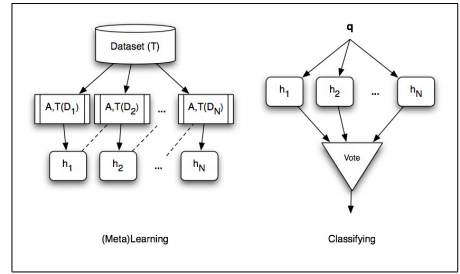2 views

How do I combine a few weak learners into a strong classifier? I know the formula, but the problem is that in every paper about AdaBoost that I've read there are only formulas without any example. I mean - I got weak learners and their weights, so I can do what the formula tells me to do (multiply learner by its weight and add another one multiplied by its weight and another one, etc.) but how exactly do I do that? My weak learners are decision stumps. They got attribute and threshold, so what do I multiply?

by (108k points)

A learner is called weak if it frequently induces models whose performance is only slightly better than random. Boosting is based on the observation that

finding many rough rules of thumb (i.e., weak learning) can be a lot easier than finding a single, highly accurate prediction rule (i.e., strong learning).

Boosting process then assumes that a weak learner can be made strong by frequently running it on various distributions Di over the training data T (i.e., varying the focus of the learner), and then combining the weak classifiers into a single composite classifier, as illustrated below: